CBSE Class 10 Sample Paper for 2019 Boards

Class 10
Solutions of Sample Papers for Class 10 Boards

Question 20

Find the area of the minor segment of a circle of radius 42 cm, if length of the corresponding arc is 44 cm.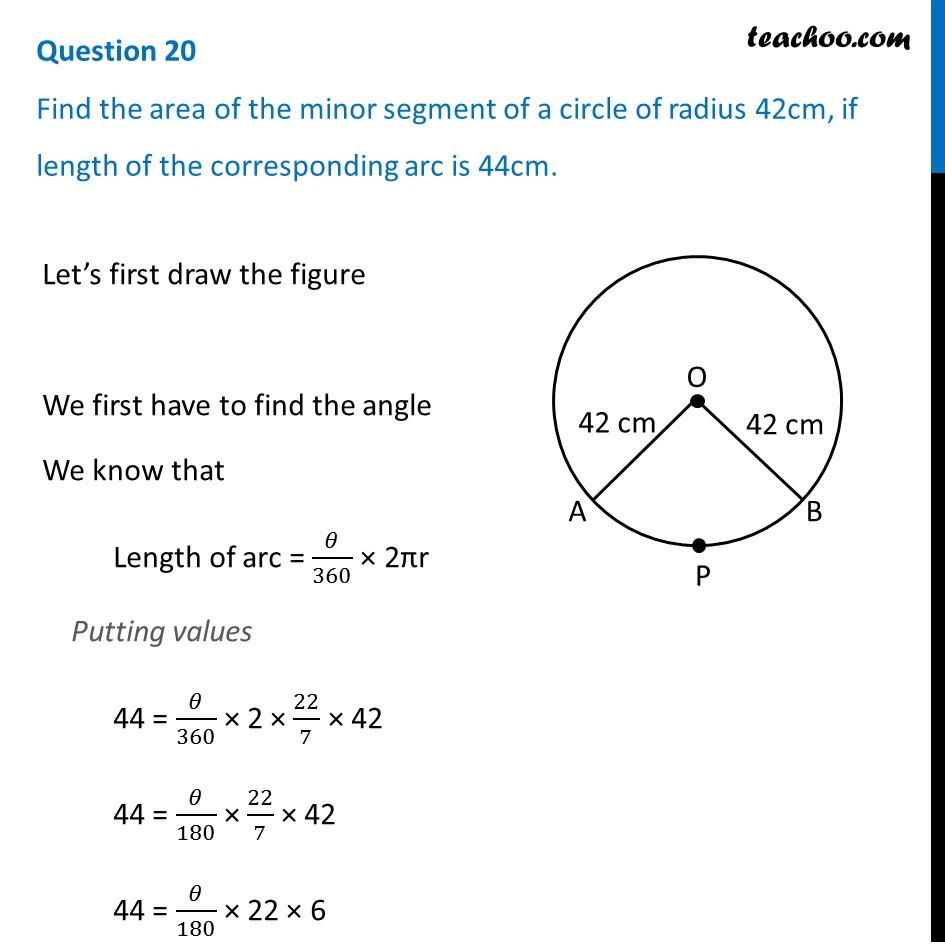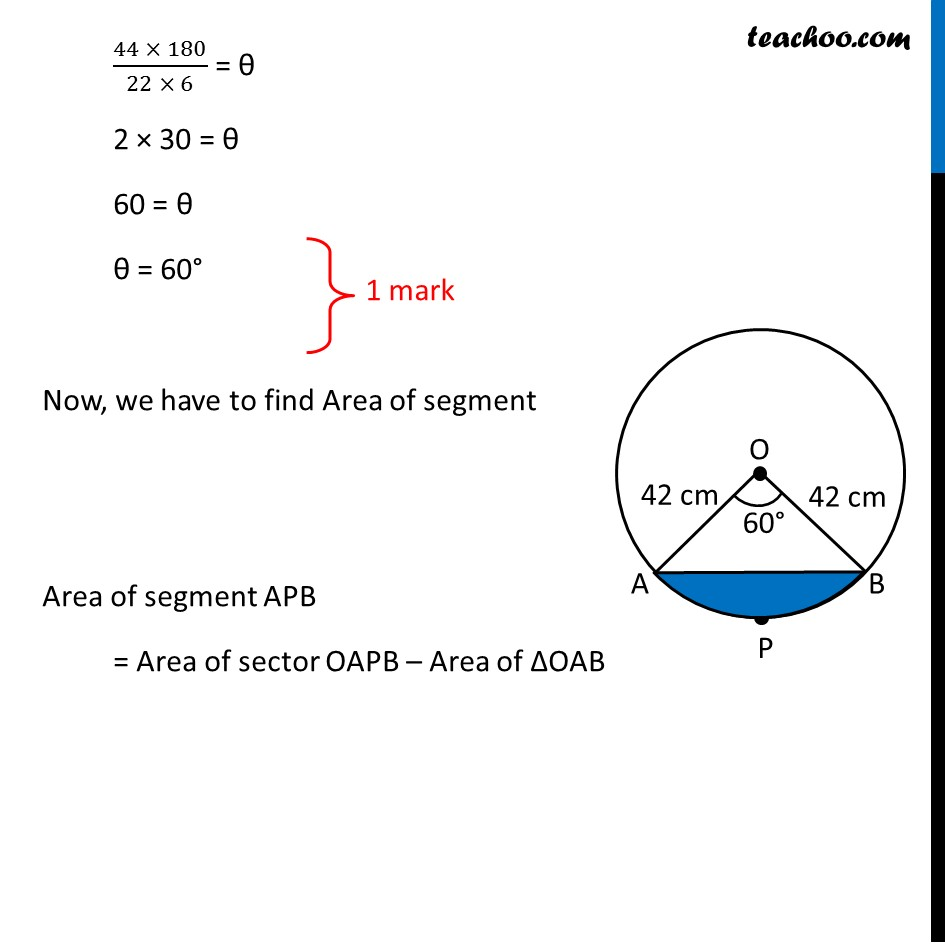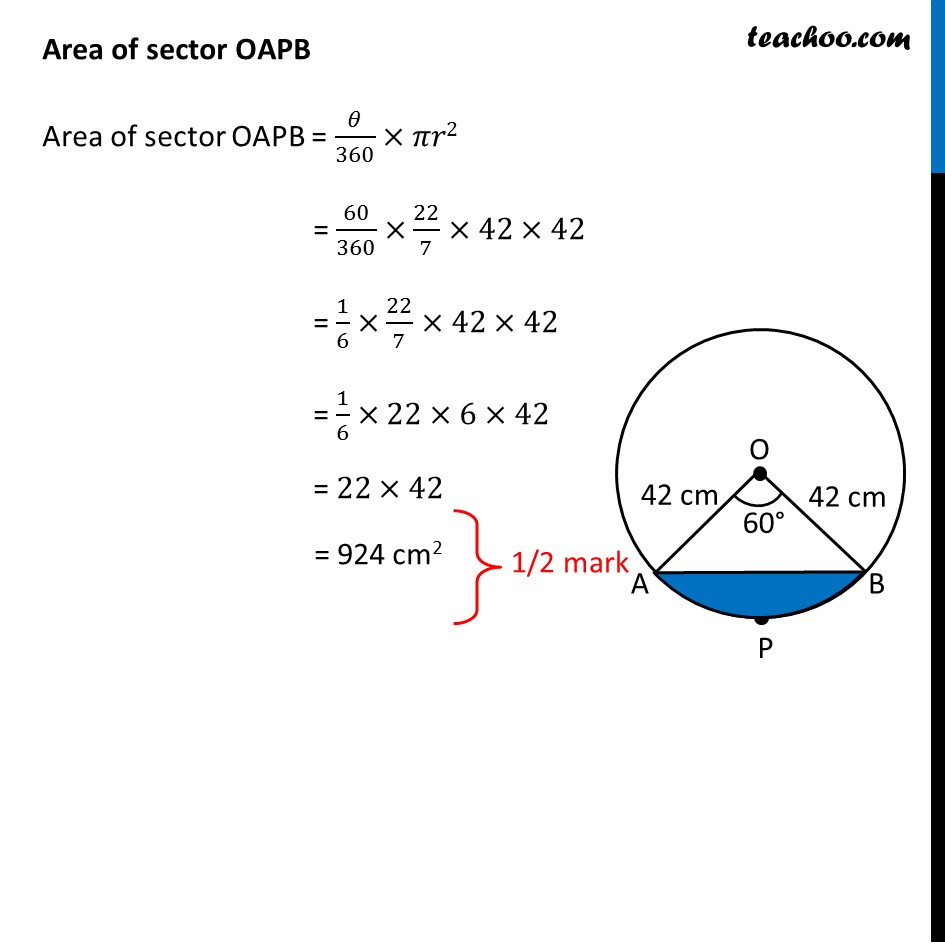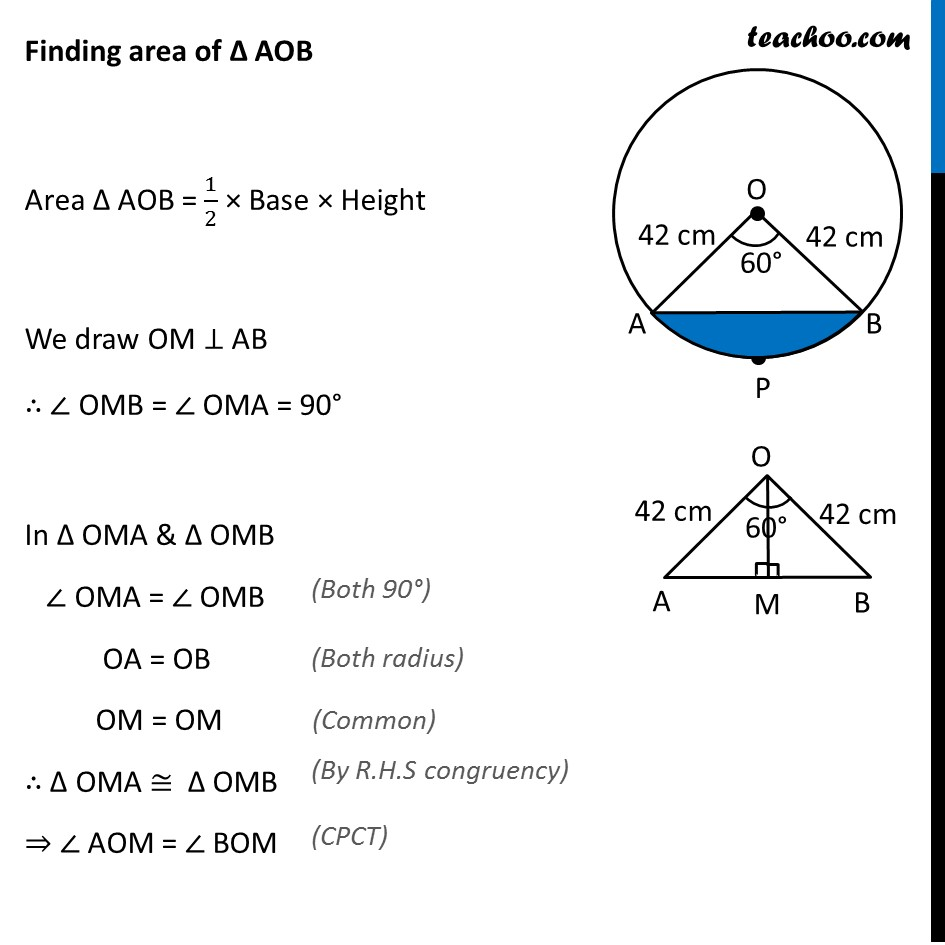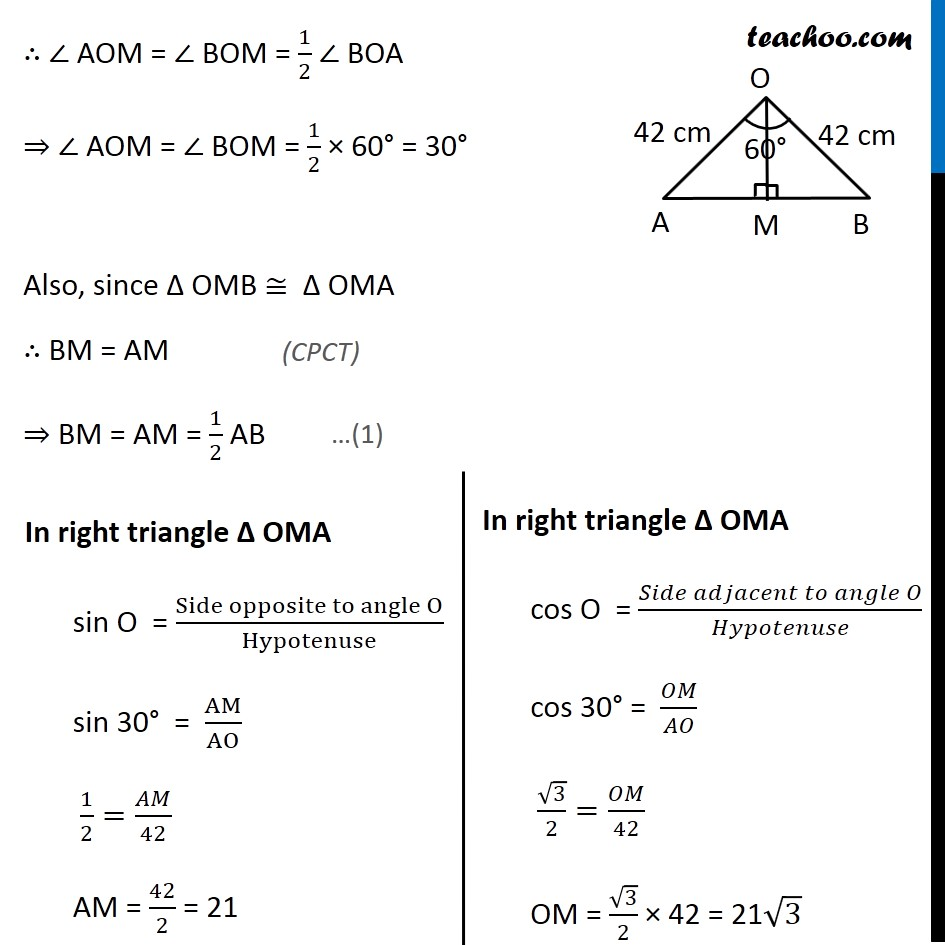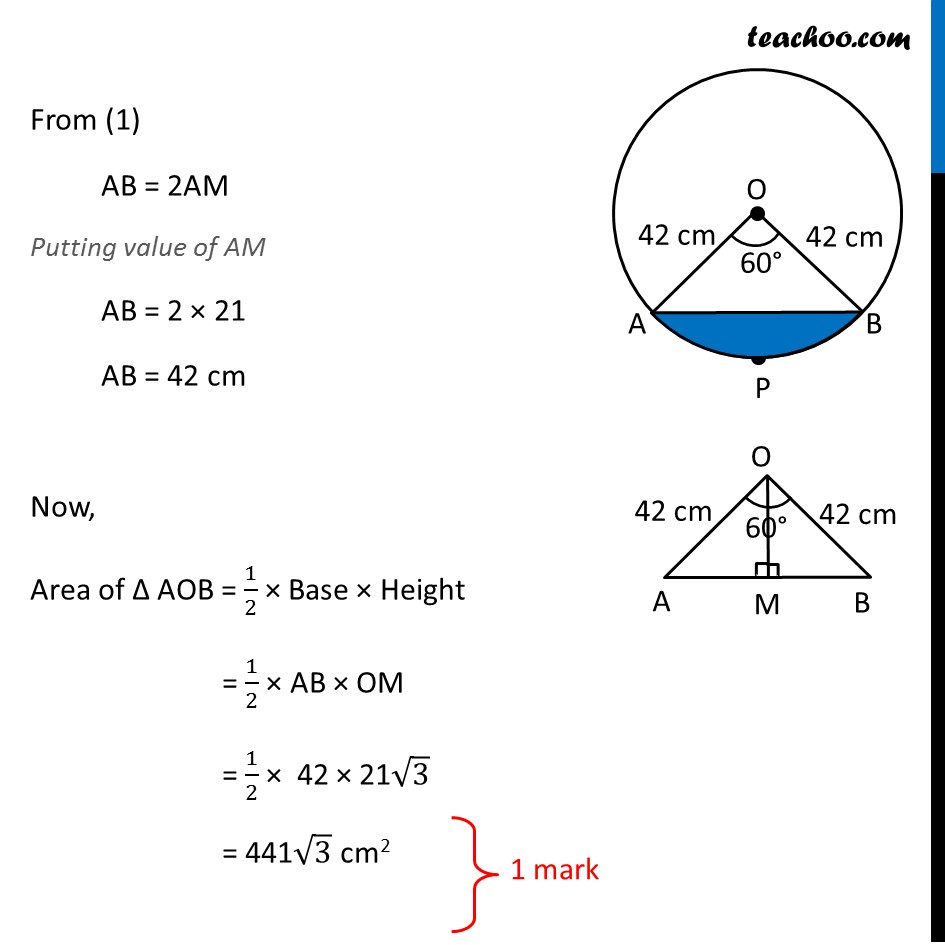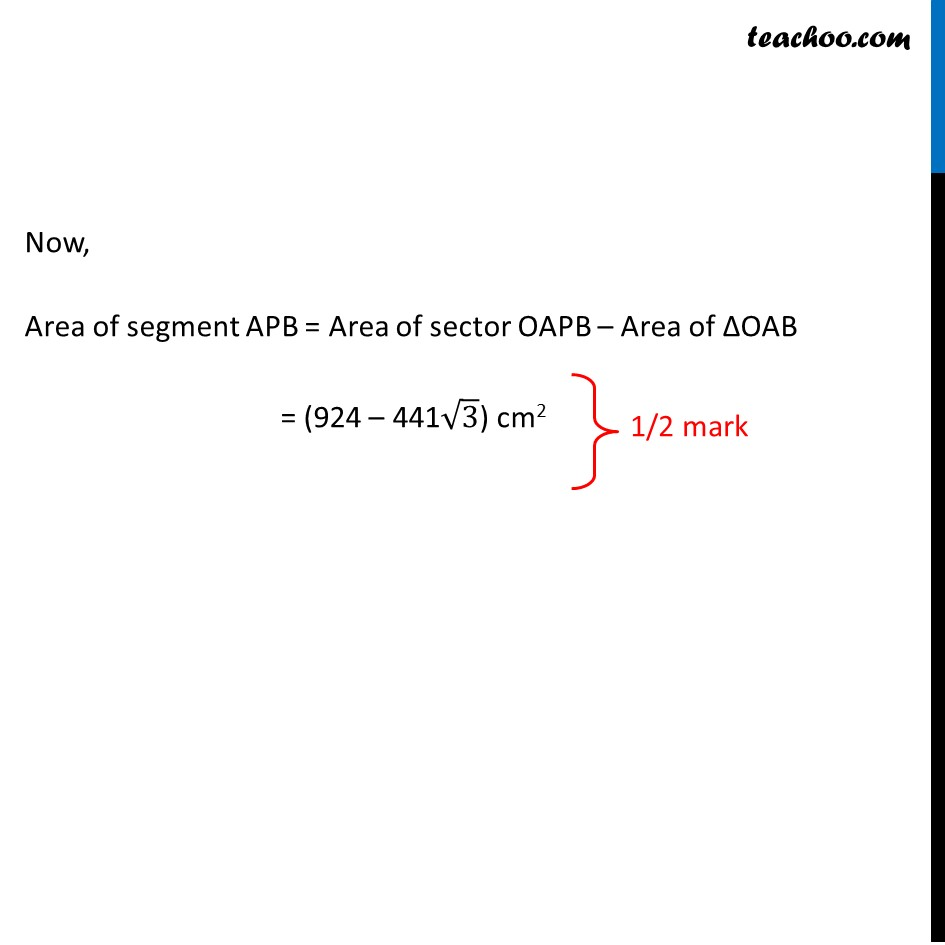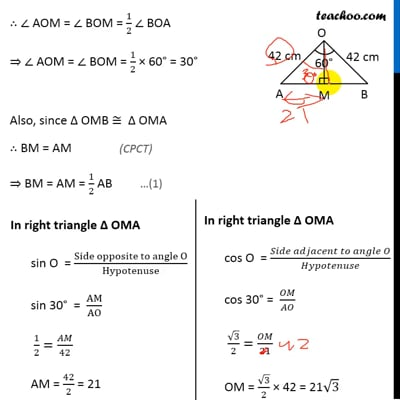This video is only available for Teachoo black users

Learn in your speed, with individual attention - Teachoo Maths 1-on-1 Class

### Transcript

Question 20 Find the area of the minor segment of a circle of radius 42cm, if length of the corresponding arc is 44cm. Let’s first draw the figure We first have to find the angle We know that Length of arc = 𝜃/360 × 2πr Putting values 44 = 𝜃/360 × 2 × 22/7 × 42 44 = 𝜃/180 × 22/7 × 42 44 = 𝜃/180 × 22 × 6 (44 × 180)/(22 × 6) = θ 2 × 30 = θ 60 = θ θ = 60° Now, we have to find Area of segment Area of segment APB = Area of sector OAPB – Area of ΔOAB Area of sector OAPB Area of sector OAPB = 𝜃/360×𝜋𝑟2 = 60/360×22/7×42×42 = 1/6×22/7×42×42 = 1/6×22×6×42 = 22×42 = 924 cm2 Finding area of Δ AOB Area Δ AOB = 1/2 × Base × Height We draw OM ⊥ AB ∴ ∠ OMB = ∠ OMA = 90° In Δ OMA & Δ OMB ∠ OMA = ∠ OMB (Both 90°) OA = OB (Both radius) OM = OM (Common) ∴ Δ OMA ≅ Δ OMB (By R.H.S congruency) ⇒ ∠ AOM = ∠ BOM (CPCT) ∴ ∠ AOM = ∠ BOM = 1/2 ∠ BOA ⇒ ∠ AOM = ∠ BOM = 1/2 × 60° = 30° Also, since Δ OMB ≅ Δ OMA (CPCT) …(1) ∴ BM = AM ⇒ BM = AM = 1/2 AB In right triangle Δ OMA sin O = (Side opposite to angle O)/Hypotenuse sin 30° = AM/AO 1/2=𝐴𝑀/42 AM = 42/2 = 21 In right triangle Δ OMA cos O = (𝑆𝑖𝑑𝑒 𝑎𝑑𝑗𝑎𝑐𝑒𝑛𝑡 𝑡𝑜 𝑎𝑛𝑔𝑙𝑒 𝑂)/𝐻𝑦𝑝𝑜𝑡𝑒𝑛𝑢𝑠𝑒 cos 30° = 𝑂𝑀/𝐴𝑂 √3/2=𝑂𝑀/21 OM = √3/2 × 42 = 21√3 From (1) AB = 2AM Putting value of AM AB = 2 × 21 AB = 42 cm Now, Area of Δ AOB = 1/2 × Base × Height = 1/2 × AB × OM = 1/2 × 42 × 21√3 = 441√3 cm2 Now, Area of segment APB = Area of sector OAPB – Area of ΔOAB = (924 – 441√3) cm2